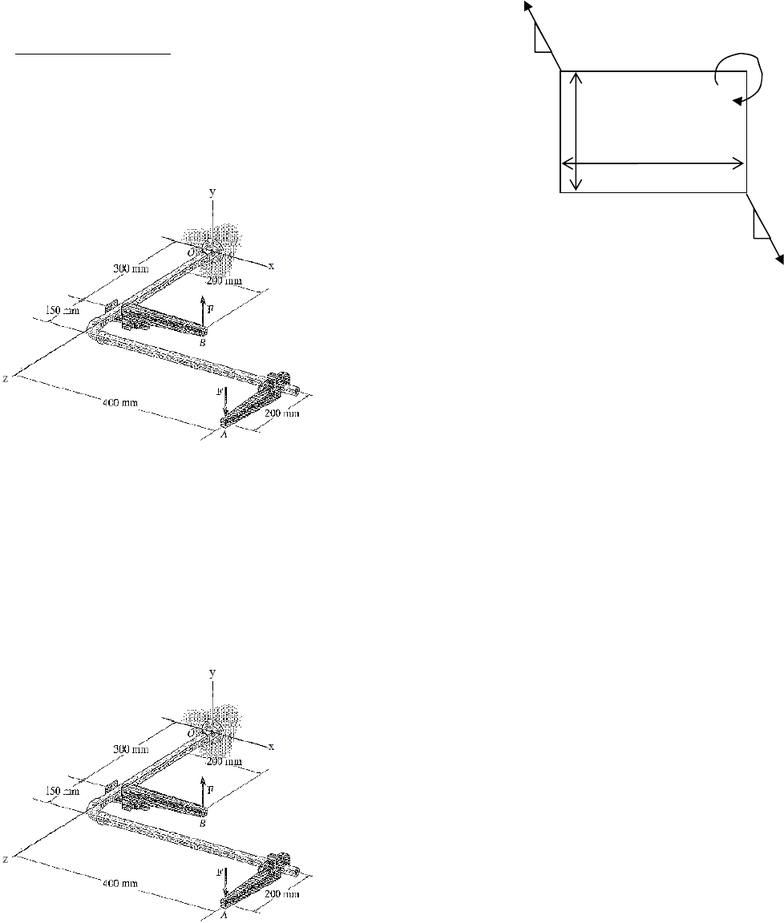# ENGG 202 Lecture Notes - Lecture 1: Unit Vector, Cross Product, Harmonic Oscillator

59 views4 pagesENGG 202 Winter 2013 Tutorial 6 February 12/13, 2013
10 N-m
2 m
4 m
30 N
30 N
5
12
5
12 AB
C
D
Moment of a couple
1) The two couples act on a rectangular plate as shown
below. a) Determine the single equivalent couple acting
on the plate. b) Determine the direction and magnitude
of a horizontal force exerted at B so that the resultant
moment of all the forces about point D is zero.
ANS: a) M =
97.7 N-m CW,
b) FB = 48.8 N
left
Solution Strategy: part a) 1 couple is shown as a pair of
forces, the other is represented by its moment. To find
the single equivalent couple (or moment) acting on the
plate, we simply need the total moment acting on the
plate since the sum of the forces will be zero (only
couples acting on it). So calculate the moment of the 30
N couple either by summing moments about a point, or
by determining the perpendicular distance between the
two lines of action and then multiplying by the force magnitude, or by resolving each 30 N force
into x and y components (giving you two couples to replace the one) and calculating the moments
due to each couple (x comps and y comps) and adding them.
Part b) draw a horizontal force at B, (assume the direction right or left as you wish). Then sum
the moments about point D to find the magnitude of this force. Since you already calculated the
total moment due to the couples in part a) then the sum of moments (which must equal 0) is the
moment due to the force you added at B plus the total moment from part a) (couples are free
vectors so the moment must be applied everywhere and is the same everywhere)
2) What is the magnitude of the forces that must be
applied at A and B if the moment of the couple must
have a magnitude of 600 N-m. ANS: 1488.4N
Solution Strategy: You can use vectors to solve: |rAB x F| = 600 Nm, or use the scalar definition
of the moment for the couple. The diagonal distance AB is the distance that is perpendicular to
both lines of action (vertical lines) so
600 = Fd, calc the diagonal distance d and you can solve for F.
Unlock document

This preview shows page 1 of the document.
Unlock all 4 pages and 3 million more documents.